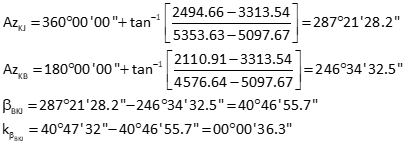### Article Index

Given the network and data in Figure F-5, determine:

• adjusted coordinates of points J and K.
• their standard errors
• their standard errorsFigure F-5 Combined Observations

There are seven observations and four unknowns: NJ, EJ, NK, EK.  The network has 7-4 = 3 DF.

### a. Matrix structures

Matrix structures are:### b. Initial approximations for points K and J

The computations are summarized and results shown:

• Distance and direction for line BA by inverse
• Solve angle ABK using Law of Cosines: 28°28'09"
• Compute direction of BK using direction BA and angle ABK: 66°33'27"
• Use direction BK and 1309.94' to compute coordinates of K: 5097.77' N, 3312.73' E
• Solve angle JBA using Law of Cosines: 11°56'48"
• Compute direction of BJ using direction BA and angle JBA: 26°08'30"
• Use direction BJ and 871.35' to compute coordinates of J: 5358.86' N, 2494.82' E

### c. Compute weight matrix### d. Distances

The distances are computed from the fixed coordinates of A and B and the initial approximations of J and K.### e. Build [C] and [K] matrices

Note: it is extremely important to minimize rounding errors by carrying enough digits in computations. Not doing so can either increase the number of iterations needed for a solution or could cause the solution to diverge.

#### (1) Distance observations

Obs 1: Line KBObs 2: Line KJObs 3: Line KAObs 4: Line JAObs 5: Line JB#### (2) Angle observations

Obs 6: Angle BKJObs 7: Angle JKA#### (3) Assemble [C] and [K] matrices### f. Solve U=[Q] x [CTWK]

Rather than detail the complete solution process, the mtrices and partial products are shown for each iteration.

#### (1) First IterationThe corrections aren't small enough so update the coordinates and repeat solution.

Updated coordinates:Repeat as corrections are not small enough.

#### (2) Second Iteration

Using the updated coordinates, go back to Step d, recompute distances and matrices, and solve for [U]The corrections still aren't small enough so update the coordinates and repeat solution.

Updated coordinates:#### (3) Third IterationCorrections are acceptably small enough. Update coordinates one last time.### g. Compute statistics

#### (1) Compute So and adjusted point uncertainties

Using last [C], [K] and [U] matricies, determine residuals from [V] = [C] x [U] - [K]

Use [V] and [W] to compute So , then So and [Q] to compute standard deviations of the adjusted coordinates.Add residuals to the original observationsCompute standard errors for the adjusted observations. Example comps are shown for distance KB and angle BKJ.

Obs 1: distance KB

Use first row of [C] and first column of [CT].Obs 6: Angle BKJ

Use sixth row of [C] and sixth column of [CT].Remaining standard errors are included in the following section.